Home | | Maths 12th Std | Evaluation of a Bounded Plane Area by Integration

# Evaluation of a Bounded Plane Area by Integration

In the present section, we apply the approach for finding areas of plane regions bounded by plane curves.

Evaluation of a Bounded Plane Area by Integration

In the beginning of this chapter, we have already introduced definite integral by a geometrical approach. In that approach, we have noted that, whenever the integrand of the definite integral is non-negative, the definite integral yields the geometrical area. In the present section, we apply the approach for finding areas of plane regions bounded by plane curves.

## 1. Area of the region bounded by a curve, x ŌĆō axis and the lines x = a and x = b.

Case (i)

Let y = f ( x ), a Ōēż x Ōēż b be the equation of the portion of the continuous curve that lies above the x ŌłÆ axis (that is, the portion lies either in the first quadrant or in the second quadrant) between the lines x = a and x = b . See Fig.9.8. Then, y Ōēź 0 for every point of the portion of the curve. Consider the region bounded by the curve, x ŌłÆ axis, the ordinates x = a and x = b . It is important to note that y does not change its sign in the region. Then, the area A of the region is found as follows:Viewing in the positive direction of the y ŌłÆ axis, divide the region into elementary vertical strips (thin rectangles) of height y and width ╬öx . Then, A is the limit sum of the areas of the vertical strips.

Hence, we getCase (ii)

Let y = f ( x ), a Ōēż x Ōēż b be the equation of the portion of the continuous curve that lies below the x ŌłÆ axis (that is, the portion lies either in the third quadrant or in the fourth quadrant). Then, y Ōēż 0 for every point of the portion of the curve. It is important to note that y does not change its sign in the region. Consider the region bounded by the curve, x ŌłÆ axis, the ordinates x = a and x = b . See Fig.9.9. Then, the area A of the region is found as follows:Viewing in the negative direction of the y ŌłÆ axis, divide the region into elementary vertical strips (thin rectangles) of height |y| = ŌłÆ y and width ╬öx . Then, A is the limit of the sum of the areas of the vertical strips. Hence, we get

A = lim ŌłæaŌēżxŌēżb ŌłÆy╬öx = ŌłÆbŌł½a ydx = | bŌł½a ydx | .Case (iii)

Let y = f ( x ), a Ōēż x Ōēż b be the equation of the portion of the continuous curve that lies above as well as below the x ŌłÆ axis (that is, the portion may lie in all quadrants).

Draw the graph of y = f ( x) in the XY ŌłÆ plane. The graph lies alternately above and below the x ŌłÆ axis and it is intercepted between the ordinates x = a and x = b.

Divide the interval[a , b] into subintervals [a , c1 ] , [c1 , c2 ] , . . . , [ck , b] such that f ( x) has the same sign on each of subintervals. Applying cases (i) and (ii), we can obtain individually, the geometrical areas of the regions corresponding to the subintervals.Hence the geometrical area of the region bounded by the graph of y = f ( x) , the x-axis, the lines x = a and x = b is given byFor instance, consider the shaded region in Fig. 9.10. Here A1 , A2 , A3 , and A4 denote geometric areas of the individual parts. Then, the total area is given by## 2. Area of the region bounded by a curve, yŌĆō axis and the lines y = c and y = d.

Case (iv)

Let x = f ( y ), c Ōēż y Ōēż d be the equation of the portion of the continuous curve that lies to the right side of y ŌłÆ axis (that is, the portion lies either in the first quadrant or in the fourth quadrant). Then, x Ōēź 0 for every point of the portion of the curve. It is important to note that x does not change its sign in the region.Consider the region bounded by the curve, y ŌłÆ axis, the lines y = c and y = d . The region is sketched as in Fig. 9.11. Then, the area A of the region is found as follows:

Viewing in the positive direction of the x ŌłÆ axis, divide the region into thin horizontal strips (thin rectangles) of length x and width ╬öy . Then, A is the limit of the sum of the areas of the horizontal strips. Hence, we get A = lim ŌłæcŌēżyŌēżd x╬ö y = dŌł½c xdy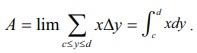Case (v)

Let x = f ( y ), c Ōēż y Ōēż d be the equation of the portion of the continuous curve that lies to the left side of y ŌłÆ axis (that is, the portion lies either in the second quadrant or in the third quadrant). Then, x Ōēż 0 for every point of the portion of the curve. It is important to note that x does not change its sign in  the region. Consider the region bounded by the curve, y ŌłÆ axis, the lines y = c and y = d . The region is sketched as in Fig. 9.12. Then, the area A of the region is found as follows:Viewing in the positive direction of the x ŌłÆ axis, divide the region into thin horizontal strips (thin rectangles) of length |x| = ŌłÆx and width ╬öy . Then, A is the limit of the sum of the areas of the horizontal strips.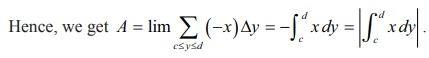Case (vi)

Let x = f ( y ), c Ōēż y Ōēż d be the equation of the portion of the continuous curve that lies to the right as well as to the left of the y ŌłÆ axis (that is, the portion may lie in all quadrants). Draw the graph of x = f ( y) in the XY ŌłÆ plane. The graph lies alternately to the right and to the left of the y ŌłÆ axis and it is intercepted between the lines y = c and y = d . Divide the interval [c , d ] into subintervals [c , a1 ] , [a1 , a2 ] , . . . , [ak , d ] such that f ( y) has the same sign on each of subintervals. Applying cases (iii) and (iv), we can obtain individually, the geometrical areas of the regions corresponding to the subintervals.Hence the geometrical area A of the region bounded by the graph of x = f ( y) , the y-axis, the lines y = c and y = d is given byFor instance, consider the shaded region in Fig. 9.13. Here, B1 , B2 , B3 and B4 denote geometric areas of the individual parts. Then the total area B of the region bounded by the curve x = f(y), y ŌłÆ axis and the lines y = c and y = d is given by

B = B1+B2+B3+B4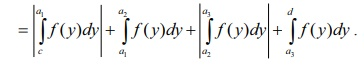Example 9.47

Find the area of the region bounded by the line 6x + 5y = 30 , x ŌłÆ axis and the lines x = ŌłÆ1 and x = 3 .

Solution

The region is sketched in Fig. 9.14. It lies above the x ŌłÆ axis. Hence, the required area is given byExample 9.48

Find the area of the region bounded by the line 7x ŌłÆ 5y = 35 , x ŌłÆ axis and the lines x = ŌłÆ2 and x = 3.

Solution

The region is sketched in Fig. 9.15. It lies below the x ŌłÆ axis. Hence, the required area is given byExample 9.49

Find the area of the region bounded by the ellipse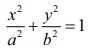Solution

The ellipse is symmetric about both major and minor axes. It is sketched as in Fig.9.16. So, viewing in the positive direction of y -axis, the required area A is four times the area of the region bounded by the portion of the ellipse in the first quadrantNote

Viewing in the positive direction of x -axis, the required y  area A is four times the area of the region bounded by the portion of the ellipse in the first quadranty-axis, y = 0 and y = b. Hence, by taking horizontal strips (see Fig.9.17), we get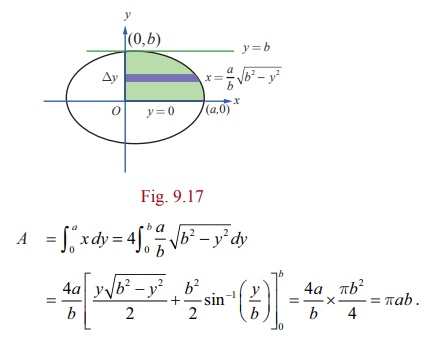Note

Putting b = a in the above result, we get that the area of the region enclosed by the circle x2+ y2 = a2 is ŽĆa2 .

Example 9.50

Find the area of the region bounded between the parabola y2 = 4ax and its latus rectum.

Solution

The equation of the latus-rectum is x = a . It intersects the parabola at  the points L ( a, 2a) and L1 ( a, ŌłÆ2a) . The required area is sketched in Fig. 9.18. By symmetry, the required area A is twice the area bounded by the portion of the parabola

y = 2 ŌłÜaŌłÜx , x -axis, x = 0 and x = a.Hence, by taking vertical strips, we getNote

Viewing in the positive direction of x-axis, and making horizontal strips (see Fig. 9.19), we get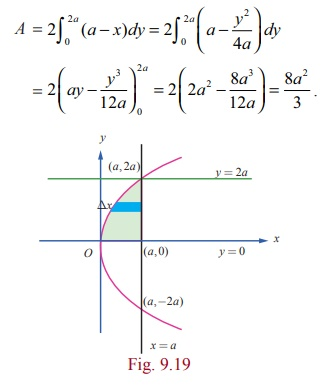Note

It is quite interesting to note that the above area is equal to two-thirds the base (latus-rectum) times the height (the distance between the focus and the vertex). This verifies ArchimedesŌĆÖ formula for areas of parabolic arches which states that the area under a parabolic arch is two-thirds the area of the rectangle having base of the arch as length and height of the arch as the breadth. It is also equal to four-thirds the area of the triangle with base (latus-rectum) and height (the distance between the focus and the vertex).

Example 9.51

Find the area of the region bounded by the y -axis and the parabola x = 5 ŌłÆ 4 y ŌłÆ y2.

Solution

The equation of the parabola is ( y + 2)2 = ŌłÆ ( x ŌłÆ 9) . The parabola crosses the y -axis at (0, ŌłÆ5) and (0,1) .The vertex is at (9, ŌłÆ2) and the axis of the parabola is y = ŌłÆ2 . The required area is sketched as in Fig. 9.20.Viewing in the positive direction of x ŌłÆ axis, and making horizontal strips, the required area A is given by### Note

As in the previous problem, we again verify ArchimedesŌĆÖ formula that the area of the parabolic arch is equal to two-thirds the base times the height.

### Example 9.52

Find the area of the region bounded by x ŌłÆ axis, the sine curve y = sin x , the lines x = 0 and x = 2ŽĆ .

### Solution

The required area is sketched in Fig. 9.21. One portion of the region lies above the x ŌłÆ axis between x = 0 and x = ŽĆ , and the other portion lies below x ŌłÆ axis between x = ŽĆ and x = 2ŽĆ .

So, the required area is given byNote

If we compute the definite integral 2ŽĆŌł½0sin xdx , we getSo 2ŽĆŌł½0 f (x)dx does not represent the area of the region bounded by the curve y = sin x , x ŌłÆ axis, the lines x = 0 and x = 2ŽĆ .

### Example 9.53

Find the area of the region bounded by x ŌłÆ axis, the curve y = |cos x|, the lines x = 0 and x = ŽĆ .

SolutionIt lies above the x ŌłÆ axis. The required area is sketched in Fig. 9.22. So, the required area is given by## 3. Area of the region bounded between two curves

Case (i)

Let y = f ( x) and y = g( x) be the equations of two curves in the XOY ŌłÆ plane such that f (x) Ōēź g ( x) for all x Ōłł [a, b] . We want to find the area A of the  region bounded between the two curves, the ordinates x = a and  x = b .The required area is sketched in Fig. 9.23. To compute A , we divide the region into thin vertical strips of width ╬öx and height f ( x) ŌłÆ g ( x) . It is important note that f (x) ŌłÆ g (x) Ōēź 0 for all x Ōłł[a, b] . As before, the required area is the limit of the sum of the areas of the vertical strips. Hence, we get

A = bŌł½a [ f (x) - g(x)]dx

Note

Viewing in the positive direction of y ŌłÆ axis, the curve y = f ( x) can be termed as the upper curve (U) and the curve y = g( x) as the lower curve (L). Thus, we get A = bŌł½a [ yU ŌłÆ yL]dx

Case (ii)

Let x = f ( y) and x = g( y) be the equations of two curves in the XOY ŌłÆ plane such that f ( y) Ōēź g ( y) for all y Ōłł[c, d ] . We want to find the area A of the region bounded between the two curves, the lines y = c and y = d . The required area is sketched in Fig. 9.24.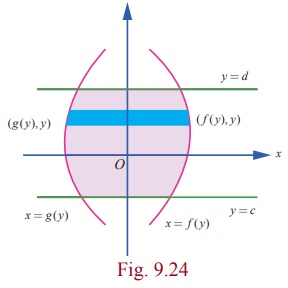To compute A , we view in the positive direction of the x ŌĆō axis and divide the region into thin horizontal strips of width ╬öy and height f ( y) ŌłÆ g ( y) . It is important note that f ( y) ŌłÆ g ( y) Ōēź 0 for all y Ōłł[c, d ] . As before, the required area is the limit of the sum of the areas of the horizontal strips. Hence, we get

A = dŌł½c [ f (x) - g(x)]dx

Note

Viewing in the positive direction of x ŌłÆ axis, the curve x = f ( y) can be termed as the right curve (R) and the curve x = f ( y) as the left curve (L). Thus, we get A = bŌł½a [ xR ŌłÆ x L ]dy .

Example 9.54

Find the area of the region bounded between the parabolas y2 = 4x and x2 = 4y.

Solution

First, we get the points of intersection of the parabolas. For this, we  solve y2 = 4x and x2 = 4y simultaneously: Eliminating y between them, we get x4 = 64x and so x = 0 and x = 4 . Then the points of intersection are (0, 0) and (4, 4) . The required region is sketched in Fig.9.25.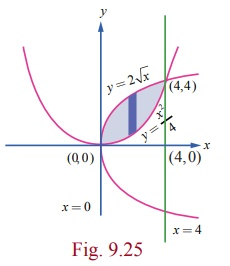Viewing in the direction of y -axis, the equation of the upper boundary is y = 2ŌłÜx for 0 Ōēż x Ōēż 4 and the equation of the lower boundary is y = x2/4 for 0 Ōēż x Ōēż 4 . So, the required area ╬ö isNote

Viewing in the positive direction of x -axis, the right bounding curve is x2 = 4y and the left bounding curve is y2 = 4x . See Fig. 9.26. The equation of the right boundary is x = 2ŌłÜy for 0 Ōēż y Ōēż 4 and the equation of the left boundary is x = y2/4 for 0 Ōēż y Ōēż 4 . So, the required area A isExample 9.55

Find the area of the region bounded between the parabola x2 = y and the curve y = |x|.

Solution

Both the curves are symmetrical about y -axis.It intersects the parabola x2 = y at ( 1,1) and ( ŌłÆ1, 1).

The area of the region bounded by the curves is sketched in Fig. 9.27. It lies in the first quadrant as well as in the second quadrant. By symmetry, the required area is twice the area in the first quadrant.

In the first quadrant, the upper curve is y  = x, 0 Ōēż x Ōēż 1 and the lower curve is y = x2, 0 Ōēż x Ōēż 1. Hence, the required area is given byExample 9.56

Find the area of the region bounded by y = cos x, y = sin x, the lines x = ŽĆ/4 and x = 5ŽĆ/4.

Solution

The region is sketched in Fig. 9.28. The upper boundary of the region is y = sin x for ŽĆ/4 Ōēż x Ōēż 5ŽĆ/4 and the lower boundary of the region is y = cos x for ŽĆ/4 Ōēż x Ōēż 5ŽĆ/4. So the required area A is given byExample 9.57

The region enclosed by the circle x2 + y2 = a2 is divided into two segments by the line x = h. Find the area of the smaller segment.

Solution

The smaller segment is sketched in Fig. 9.29. Here 0 < h < a . By symmetry about the x -axis, the area of the smaller segment is given by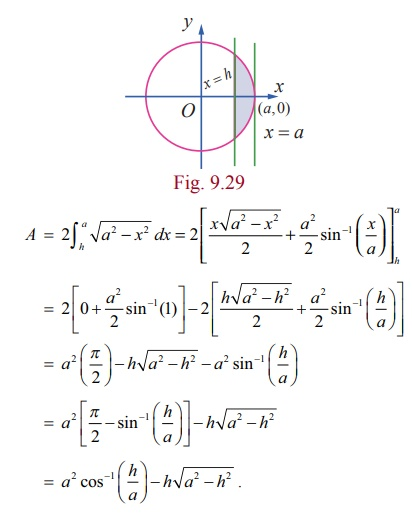Example 9.58

Find the area of the region in the first quadrant bounded by the parabola y2 = 4x , the line x + y = 3 and y -axis.

Solution

First, we find the points of intersection of x + y = 3 and y2 ŌłÆ 4x :

x + y = 3 ŌćÆ y = 3 ŌłÆ x .

Ōł┤ y 2 = 4x ŌćÆ (3 ŌłÆ x )2 = 4x

ŌćÆ x 2 ŌłÆ 10x + 9 = 0

ŌćÆ x = 1, x = 9 .Ōł┤ x =1 in x + y = 3 ŌćÆ y = 2 , and x = 9 in x + y = 3 ŌćÆ y = ŌłÆ6 .

Ōł┤(1, 2) and (9, ŌłÆ6) are the points of intersection.

The line x + y = 3 meets the y -axis at (0, 3) .

The required area is sketched in Fig. 9.30.

Viewing in the direction of y -axis, on the right bounding curve is given by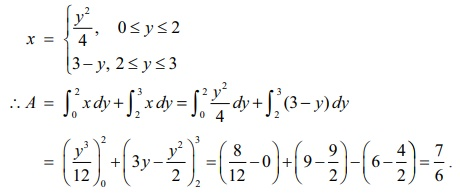Example 9.59

Find, by integration, the area of the region bounded by the lines 5x ŌłÆ 2 y = 15, x + y + 4 = 0 and the x-axis.

Solution

The lines 5x ŌłÆ 2 y = 15, x + y + 4 = 0 intersect at (1, ŌłÆ5) . The line 5x ŌłÆ 2 y = 15 meets the x-axis at ( 3, 0) . The line x + y + 4 = 0 meets the x-axis at ( ŌłÆ4, 0) . The required area is shaded in Fig.9.31. It lies below the x-axis. It can be computed either by considering vertical strips or horizontal strips.

When we do by vertical strips, the region has to be divided into two sub-regions by the line x = 1 . Then, we getWhen we do by horizontal strips, there is no need to subdivide the region. In this case, the area is bounded on the right by the line 5x ŌłÆ 2 y = 15 and on the left by x + y + 4 = 0 . So, we getNote

The region is triangular with base 7 units and height 5 units. Hence its area is 35/2  without using integration.

Example 9.60

Using integration find the area of the region bounded by triangle ABC, whose vertices A, B, and C are ( ŌłÆ1,1), ( 3, 2) , and ( 0, 5) respectively.

Solution

See Fig. 9.32.### Example 9.61

Using integration, find the area of the region which is bounded by x-axis, the tangent and normal to the circle x2 + y2 = 4 drawn at (1, ŌłÜ3) .

Solution

We recall that the equation of the tangent to the circle  x2 + y2= a2 at ( x1 , y1 ) is xx1 + yy1 = a2 . So, the equation of the tangent to the circle x2 + y2 = 4 at (1, ŌłÜ3) is x + y ŌłÜ3 = 4 ; that is, y =  ŌłÆ 1/ŌłÜ3 (x ŌłÆ 4) . The tangent meets the x-axis at the point (4,0). The slope of the tangent is ŌĆō1/ŌłÜ3. So the slope of the normal is ŌłÜ3 and hence equation of the normal is y ŌłÆ ŌłÜ3 = ŌłÜ3( x ŌłÆ1) ; that is y = ŌłÜ3x and it passes through the origin. The area to be found is shaded in the adjoining figure. It can be found by two methods.Method 1

Viewing in the postive direction of y-axis, the required area is the area of the region bounded by x-axis, y = ŌłÜ3x and x + y ŌłÜ3 = 4 . So it can be obtained by applying the formula bŌł½a ydx . For this, we have to split the region into sub-regions, one sub-region bounded by x-axis, the normal y = ŌłÜ3x and the line x = 1 ; the other sub-region bounded by x-axis, the tangent x + yŌłÜ3= 4 and the line x = 1 axis.### Method 2

Viewing in the direction of x-axis, the required area is the area of the region bounded between y = ŌłÜ3x and x + yŌłÜ3 = 4 , y = 0 and y = ŌłÜ3. So, it can be obtained by applying the formula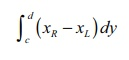Here, c = 0 , d = ŌłÜ3 , xR is the x-value on the tangent x + yŌłÜ3 = 4 and xL is the x-value on the normal y = ŌłÜ3x .### Working rule for finding area of the region bounded by y = f1(x), y = f2(x), the lines x=a and x = b , where a < b :

Draw an arbitrary line parallel to y-axis cutting the plane region. First, find the y-coordinate of the point where the line enters the region. Call it yENTRY . Next, find the y-coordinate of the point where the line exits the region. Call it yEXIT . Both yENTRY and yEXIT can be found from the equations of the bounding curves. Then, the required area is given by bŌł½a [ y EXIT ŌłÆ yENTRY ]dx.

### Working rule for finding area of the region bounded by x = g1(y), x = g2(y), the lines y=c and y = d , where c < d :

Draw an arbitrary line parallel to x-axis cutting the plane region.

First, find the x-coordinate of the point where the line enters the region. Call it xENTRY .

Next, find the x-coordinate of the point where the line exits the region. Call it xEXIT . Both xENTRY and xEXIT can be found from the equations of the bounding curves. Then, the required area is given by dŌł½c [ x EXIT ŌłÆ xENTRY ]dy .

Tags : Applications of Integration | Mathematics , 12th Maths : UNIT 9 : Applications of Integration
Study Material, Lecturing Notes, Assignment, Reference, Wiki description explanation, brief detail
12th Maths : UNIT 9 : Applications of Integration : Evaluation of a Bounded Plane Area by Integration | Applications of Integration | Mathematics